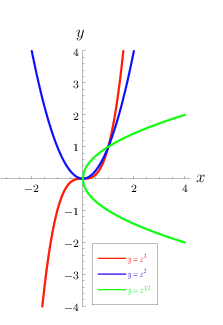# Monomial Function: Definition

## What is a Monomial Function?Power functions are a type of monomial function.A monomial function is an expression with only one term. The power function f(x) = axn is sometimes called “the” monomial function , but there are other possibilities. For example, a polynomial function is the sum of many monomial functions; If there’s only one term in a polynomial function, then it is a monomial function .

Monomial functions can take many forms:

1. A monomial (a number by itself is a “monomial”). These single-valued functions are usually called constant functions:
• y = 67,
• y = π
• y = 230,000
2. A monomial, multiplied by another monomial. For example, y = 2 * 5 = 10
3. A monomial multiplied by a constant/variable combination. For example y = 2 * 2x = 4x. If you multiply any variables (e.g. x, y, or z) along with the numbers, those are also monomials. For example:
• 2x,
• 3x2,
• 45xyz,
• 905abc,
• 75xz2.

## What isn’t a monomial?

A variable with a negative exponent is the same as having that variable in the denominator. Therefore, if you have an function that has an expression with a negative exponent, it isn’t a monomial (it’s a polynomial function).

Other examples of what isn’t a monomial function:

• 4x + 2
• 70a+ 30b – 7c3
• 45y– 42z– 3y + 4z – 1
• 10x-2

All of the above expressions are not monomial functions since they have either addition or subtraction. Or, as in the last case, there’s a negative exponent. If the exponent were changed to be positive, meaning it would look like this, 10x2, then it will be a monomial.

The addition or subtraction of two or more monomials isn’t a monomial. But if you separate their parts, you’ll get monomials. For example, the first one (4x + 2) is made of two parts, 4x and 2. Both of these by themselves will be monomials.

### Degrees of monomial function

To calculate the degree of a monomial function, sum the exponents of each variable.
Example: What is the degree of 4 * a * b2 * c2?
Solution: The degree of the constant 4 is zero (i.e. there’s no exponent). However, the degrees of the variables are:

• a = 1
• b = 2
• c = 2

So, 1 + 2 + 2 = 5.
The degree is sometimes called the order when it comes to solving series.

## References

 Real Functions. Retrieved July 13, 2021 from: https://www.et.byu.edu/~vps/ET502WWW/TABLES-PDF/F.pdf
 Main Ideas. Retrieved July 13, 2021 from: https://ximera.osu.edu/fall18calcvids/o/basicderivrules/basicderivrules/preO

CITE THIS AS:
Stephanie Glen. "Monomial Function: Definition" From StatisticsHowTo.com: Elementary Statistics for the rest of us! https://www.statisticshowto.com/monomial-function/